# 阿里云、腾讯云、百度云三家云服务器简单对比

## windows篇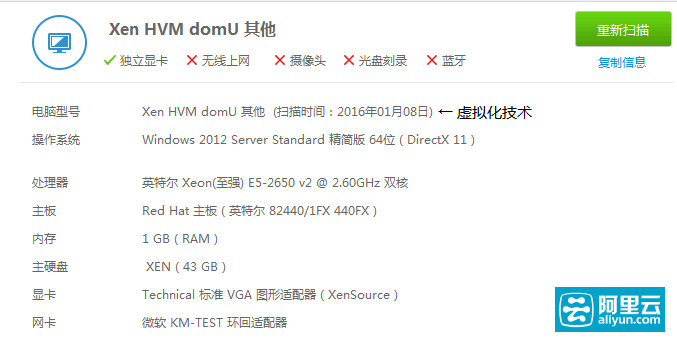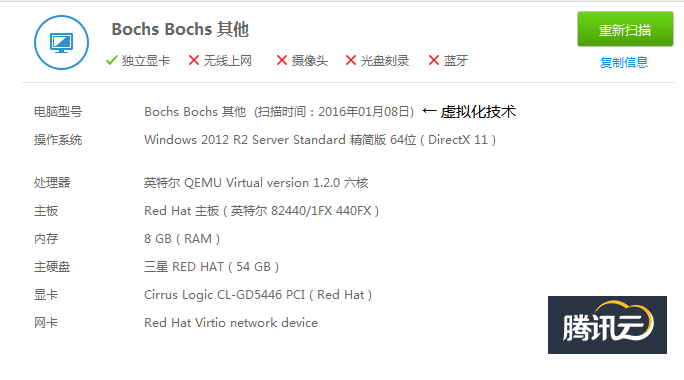CPU：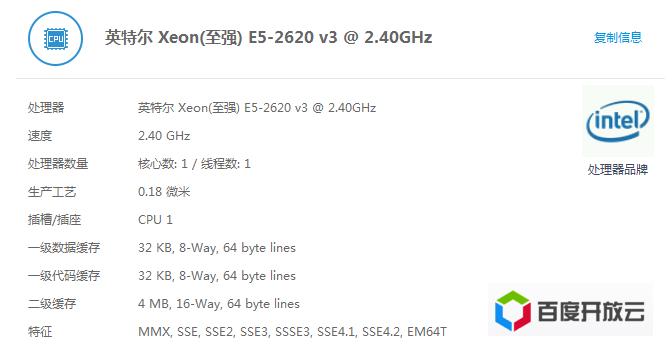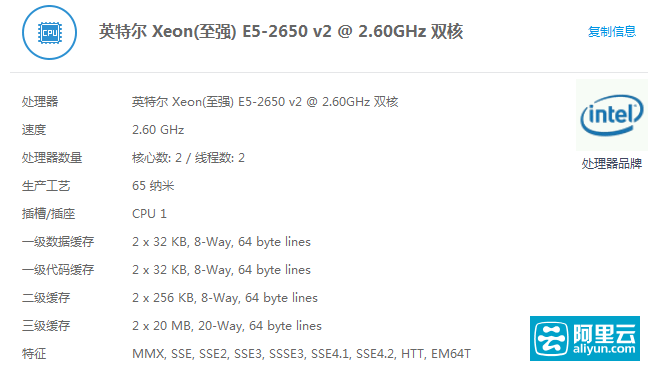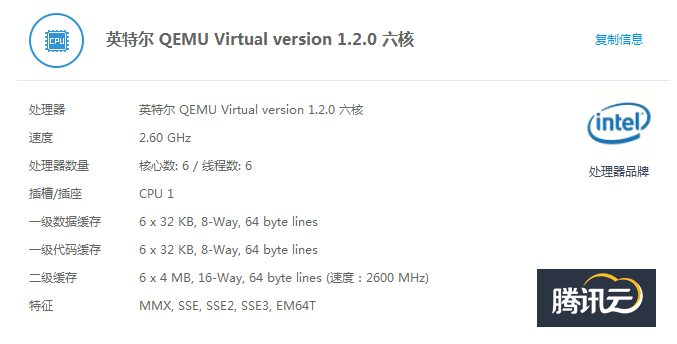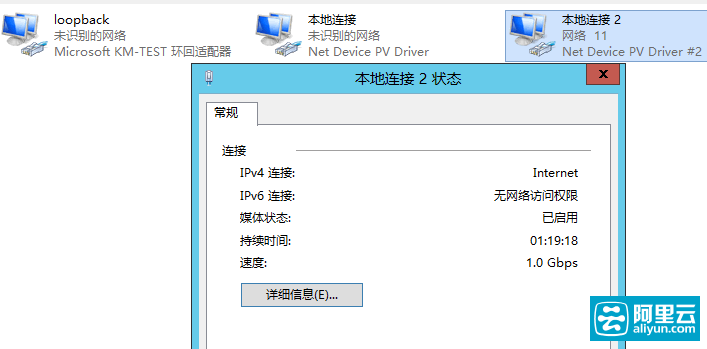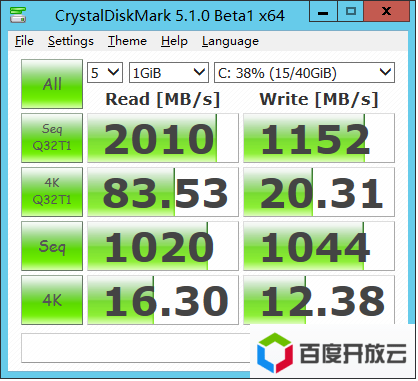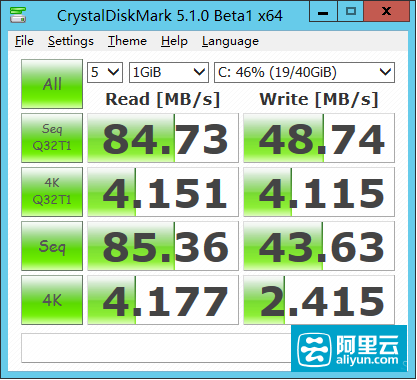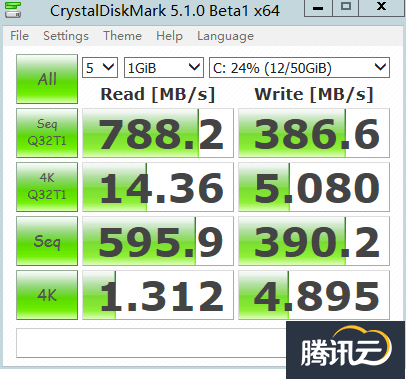## linux篇

linux就用三台云主机跑同一个脚本，看输出，脚本如下：

#!/bin/bash
#From hqidi.com
cname=\$( awk -F: '/model name/ {name=\$2} END {print name}' /proc/cpuinfo )
cores=\$( awk -F: '/model name/ {core++} END {print core}' /proc/cpuinfo )
freq=\$( awk -F: ' /cpu MHz/ {freq=\$2} END {print freq}' /proc/cpuinfo )
tram=\$( free -m | awk 'NR==2 {print \$2}' )
swap=\$( free -m | awk 'NR==4 {print \$2}' )
up=\$(uptime|awk '{ \$1=\$2=\$(NF-6)=\$(NF-5)=\$(NF-4)=\$(NF-3)=\$(NF-2)=\$(NF-1)=\$NF=""; print }')
disk=\$(fdisk -l | awk 'BEGIN {sum=0} /GB/ {sum+=\$3} END {print sum}')
device=\$(fdisk -l |awk -F'[ :]' 'NR==2 {print \$2}')
dspeed=\$(hdparm -t \$device |awk -F'=' 'END {print \$2}')
io=\$( ( dd if=/dev/zero of=test_\$\$ bs=64k count=16k conv=fdatasync && rm -f test_\$\$ ) 2>&1 | awk -F, '{io=\$NF} END { print io}' )
Virtualization=\$(virt-what)

echo "CPU model : \$cname"
echo "Number of cores : \$cores"
echo "CPU frequency : \$freq MHz"
echo "Total amount of ram : \$tram MB"
echo "Total amount of swap : \$swap MB"
echo "System uptime : \$up"
echo "Total amount of disk :\$disk GB"
echo "hdparm disk speed : \$dspeed"
echo "dd disk speed : \$io"
echo "virtualization : \$Virtualization"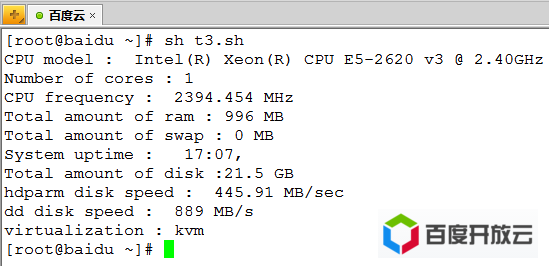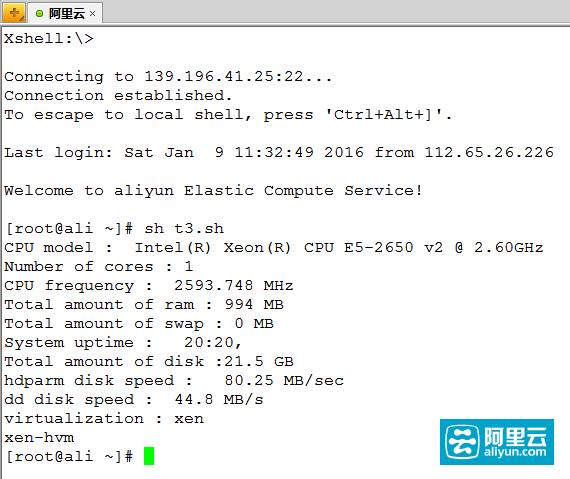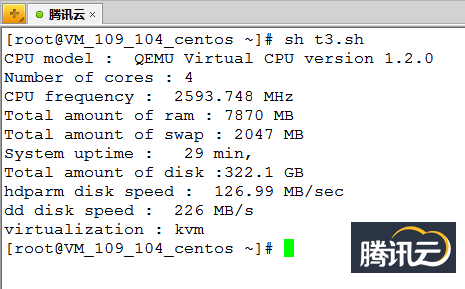## by the way

linux与windows的互换操作，实际是为您分配一台全新的云服务器

PS：百度和阿里的windows主机是可以打开音频服务，让系统发出声音的，腾讯的不行。至于为什么要云主机

### 1 条评论

•邹振忠 2017年11月27日 回复

哈哈，我用的百度云，就觉得比阿里的贵。